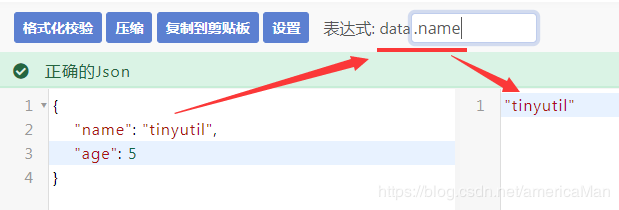### 一、什么是归并排序

#### 2.算法原理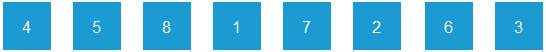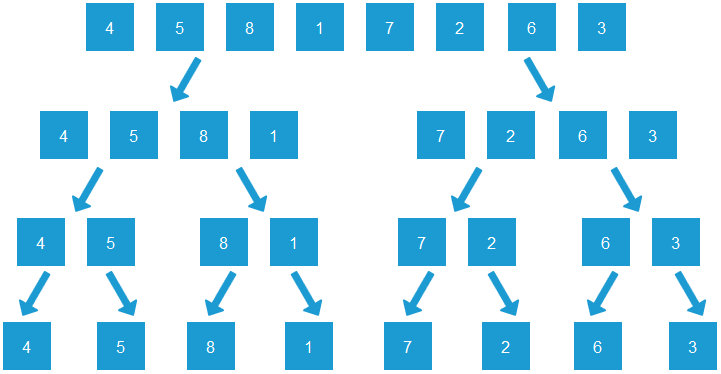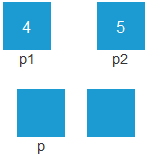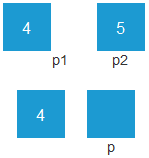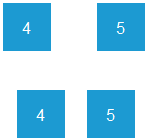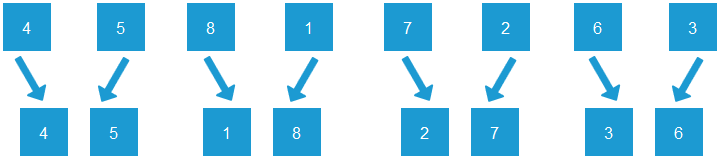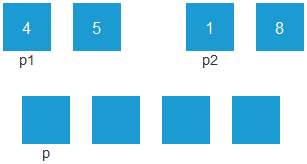4和1比较，4大于1，1填入p指向的元素，p、p2往右移一位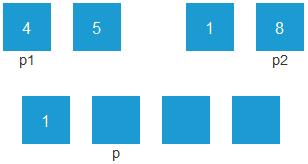4和8比较，4小于8，4填入p指向的元素，p、p1往右移一位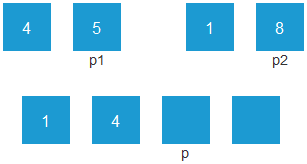5和8比较，5小于8，5填入p指向的元素，p、p1往右移一位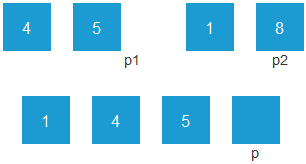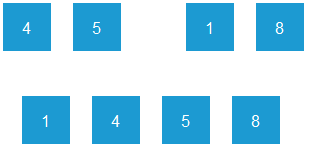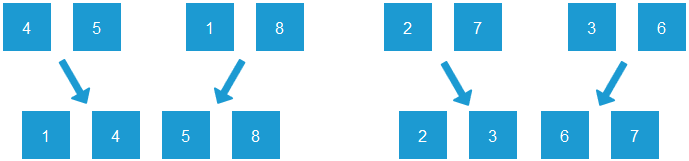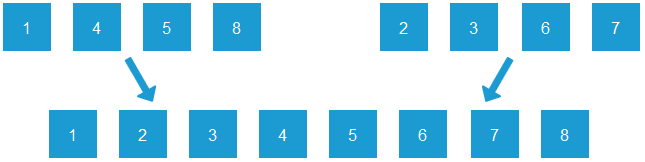#### 3.算法实现

``````function sort(arr, startIndex = 0, endIndex = arr.length - 1) {
// 递归结束条件：startIndex大于等于endIndex的时候
if (startIndex >= endIndex) {
return;
}

// 折半递归
let midIndex = parseInt((startIndex + endIndex) / 2);
sort(arr, startIndex, midIndex);
sort(arr, midIndex + 1, endIndex);
// 将两个有序的小数组，合并成一个大数组
merge(arr, startIndex, midIndex, endIndex);
}

function merge(arr, startIndex, midIndex, endIndex) {
// 新建一个大数组
let tempArr = [];
let p1 = startIndex;
let p2 = midIndex + 1;
let p = 0;

// 比较两个有序小数组的元素，依次放入大数组中
while (p1 <= midIndex && p2 <= endIndex) {
if (arr[p1] <= arr[p2]) {
tempArr[p++] = arr[p1++];
} else {
tempArr[p++] = arr[p2++];
}
}

// 右侧小数组已排序完毕，左侧小数组还有剩余，将左侧小数组元素依次放入大数组尾部
while (p1 <= midIndex) {
tempArr[p++] = arr[p1++];
}
// 左侧小数组已排序完毕，右侧小数组还有剩余，将右侧小数组元素依次放入大数组尾部
while (p2 <= endIndex) {
tempArr[p++] = arr[p2++];
}

for (let i = 0; i < tempArr.length; i++) {
arr[i + startIndex] = tempArr[i];
}
}

let arr = [4, 5, 8, 1, 7, 2, 6, 3];
sort(arr);
console.log(arr);
``````

### 二、归并排序算法特点

#### 3.稳定性

https://tinyutil.com/